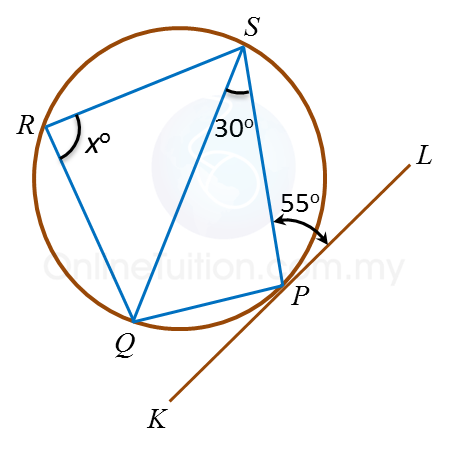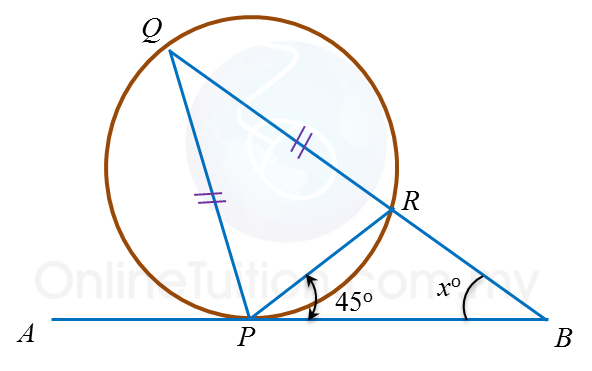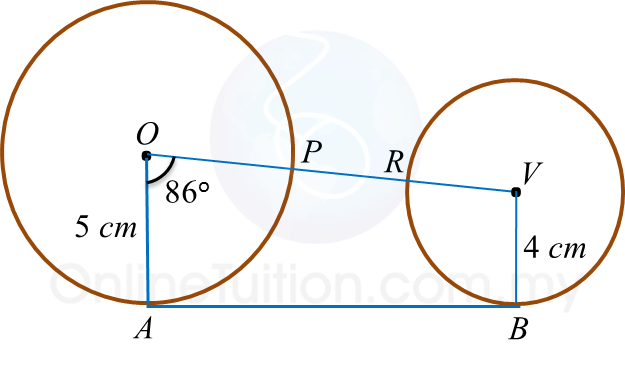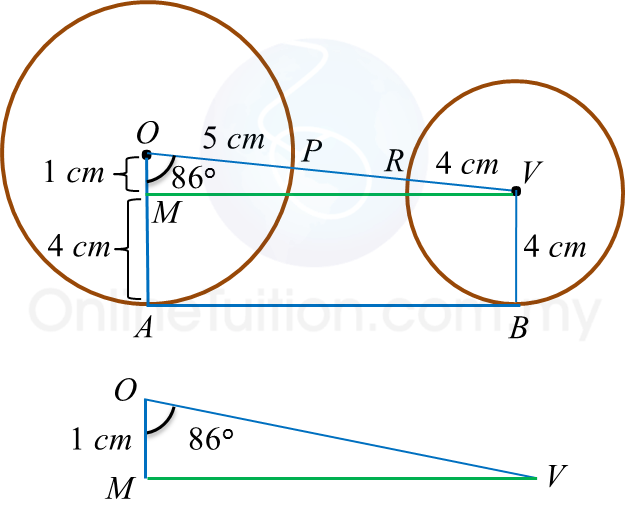# 8.4.2 Circles III, Paper 1 (Short Questions)

Question 5:In figure above, PAQ is a tangent to the circle at point A. AEC and BED are straight lines. The value of y is

Solution:
ABD = ∠ACD = 40o
ACB = ∠PAB = 60o
y= 180– ∠ACB – ∠CBD – ∠ABD
y= 180– 60o – 25o– 40o = 55o

Question 6:In figure above, KPL is a tangent to the circle PQRS at point P. The value of x is

Solution:
PQS = ∠SPL= 55o
SPQ = 180– 30o – 55o= 95o
SPQ + ∠SRQ = 180o
95o+ xo = 180o
x = 85o

Question 7:In figure above, APB is a tangent to the circle PQR at point P. QRB is a straight line. The value of x is

Solution:
PQR = ∠RPB = 45o
QPR = (180– 45o) ÷ 2 = 67.5o
PQR + ∠BPQ + xo = 180o
45+ (67.5o + 45o) + xo = 180o
x = 22.5o

Question 8:The figure above shows two circles with respective centres O and V. AB is a common tangent to the circles. OPRV is a straight line. The length, in cm, of PR is

Solution:$\begin{array}{l}\mathrm{cos}{86}^{o}=\frac{OM}{OV}\\ 0.070=\frac{1}{OV}\\ OV=\frac{1}{0.070}\\ OV=14.29cm\\ \\ \therefore PR=14.29-5-4\\ \text{}=5.29cm\end{array}$